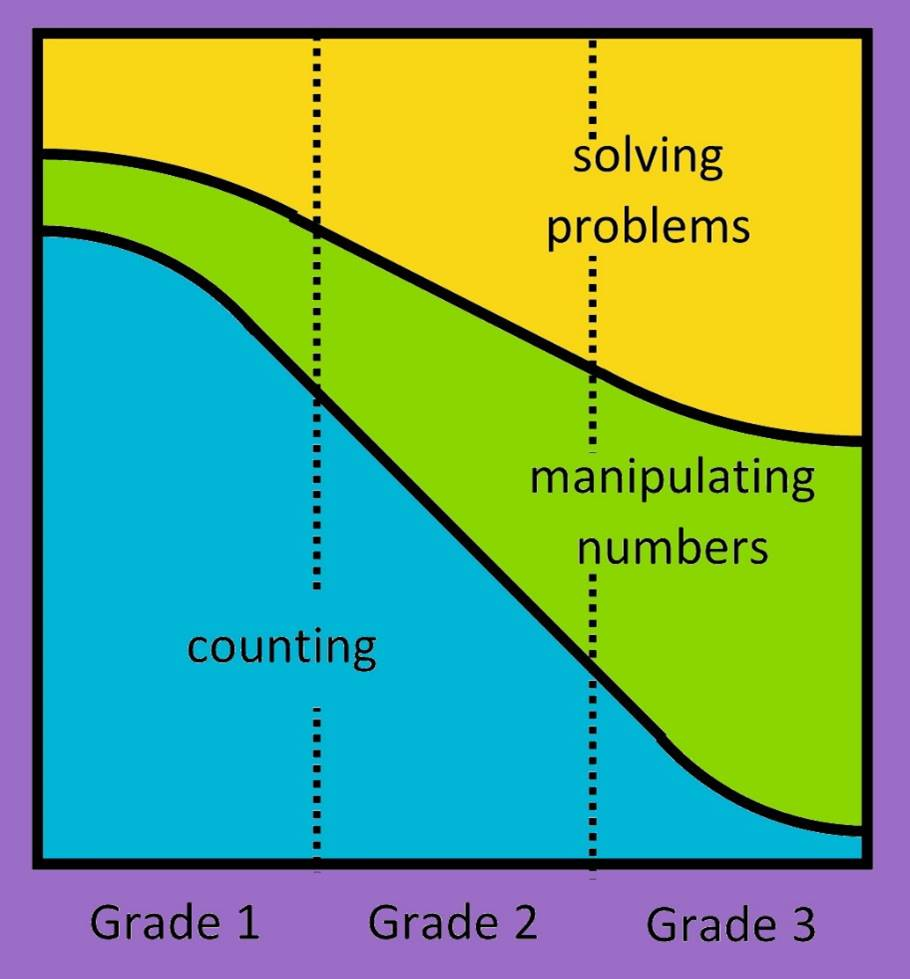• Developing Number Sense: Counting, Manipulating Numbers and Problem Solving

In the early grades, we support children’s development of number sense and progression through the stages of number development through three distinct but interrelated activities: counting, manipulating numbers and solving problems.As illustrated alongside, the amount of time that is spent on each of these activities will change over time.

Stages of number development and the NumberSense Mathematics Programme

In the NumberSense Mathematics Programme, we are mindful that children’s sense of number progresses through the three stages. The activities in the workbooks are sensitive to the stage at which the child is expected to be, while simultaneously encouraging the child to move along the developmental trajectory. That said, by the time children reach Workbook 6, they are expected to be breaking down and building up numbers within age-appropriate number ranges and by means of an increasingly larger range of strategies. These different strategies are more explicitly developed in Workbooks 13 to 24. Each page of the first twelve workbooks typically addresses the three key activities: counting, manipulating numbers and solving problems in an integrated way. The amount of counting gradually decreases in Workbooks 9 through 14.

Counting, Manipulating Numbers and Problem Solving
• The Foundation Phase Numeracy Handbook

Numeracy is an essential building block for young children to make a confident start to mathematics. The Foundation Phase Numeracy Handbook Handbook provides ideas to guide and support the teaching of Numeracy in Grades R to 3, and to plan numeracy activities that:

• Start with real problems that present children with mathematics that is presented in meaningful contexts.
• Encourage children to represent their mathematical thinking and understanding verbally and graphically.
• Involve children in a variety of dialogues which encourages them to reflect on their mathematical thinking.
Foundation Phase Numeracy Handbook
• Developing the Fraction Concept

The handouts and resources below outline and illustrate the content from a NumberSense Mathematics Programme workshop which covered the following:

• Issues that underpin a deep and meaningful understanding of fractions.
• The developmentally-appropriate sequence for teaching fractions from Grade 2 to Grade 7 used in the NumberSense Workbooks.
• Assumptions and misconceptions about the teaching of fractions.
• The role of problems in learning about fractions and experiencing mathematics as a meaningful, sense-making activity.
Developing the Fraction Concept in the Foundation Phase
The Development of the Fraction Concept Trajectory
• Developing Pre-Algebraic Thinking

The handouts and resources below outline and illustrate the content from a NumberSense Mathematics Programme workshop which covered the following:

• The role of patterning in developing number sense in particular and mathematical proficiency in general.
• An appropriate sequence (trajectory) of teaching and learning activities to support the development of patterning.
• Patterns, representations and interrelationships.
• Patterns and pre-algebra in the NumberSense classroom.
Developing the Function Concept (Patterns and Patterning) in the Primary School
The Development of Algebraic Thinking Trajectory
• Developing Geometric Thinking

The handouts and resources below outline and illustrate the content from a NumberSense Mathematics Programme workshop which covered the following:

• Developing geometric thinking in a NumberSense classroom with a focus on understanding, application and reasoning.
• Van Hiele’s levels of geometric thinking and phases of learning.
• An exciting and innovative range of interactive and grade-appropriate NumberSense geometry activities.
Developing Geometric Thinking in the Primary School
Learning through Play (Pierre Van Hiele)
• Assessment in a NumberSense Classroom

The handout below outlines the content from a NumberSense Mathematics Programme workshop which covered the following:

• Developing an annual assessment programme and appropriate assessment tasks for the NumberSense classroom.
NumberSense Assessment Resources
• Making the most of the NumberSense Workbooks

The handout below outlines the content from a NumberSense Mathematics Programme workshop which covered the following:

• Planning and executing mathematics lessons using the NumberSense Mathematics Programme.
• Classroom organisation and lesson delivery.
• Teaching mathematics for understanding, application and reasoning.
Making the most of the NumberSense Workbooks